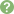Updated: 8/7/2022

# Rates

0%
Topic
Review Topic
0
0
Topic
 Introduction Overview rates measure event frequencies in a defined population during a specific time period rates are helpful in comparing event frequency in various places or times with varying population sizes Mortality Rates Crude mortality rate describes the total number of deaths per years per 1,000 people equation number of deaths during a certain time interval/mid-interval population size e.g., number of deaths in a year from January-January/population size on June 30th Cause-specific mortality rate describes the mortality rate caused by a certain disease or cause e.g., aggressive malignancy equation number of deaths by a certain disease during a certain time interval/mid-interval population Infectious Disease Rates Attack ratea type of incidence measure that is usually used in outbreak epidemiology e.g., gastrointestinal infection in patients caused by contaminated food equation number of patients with the disease during a certain time interval/total population at risk during that interval Case-fatality rateequation number of deaths by a certain disease during a certain time interval/total population affected by the disease during that interval Reproductive Rates Crude birth rate equation number of live births/total populations size Maternal mortality rate equation number of maternal deaths during a certain time interval/number of live births during that interval Neonatal mortality rate equation number of deaths of children < 28 days old during a certain time interval/number of live births during the same time interval Infant mortality rate equation number of deaths among children < 1 year of age during a certain interval/number of live births during that interval similarly, can calculate under 5 mortality rate as well Rate Standardization Rate standardization involves adjusting rates to allow for a comparison of frequency in populations with differing characteristics that may confound the results e.g., if disease X is more common in older people and population A has a higher proportion of older folks in their population, without adjustment to account for these age differences, it may appear that individuals in population A are at higher risk of a disease when in fact a population-level factor (age structure) is confounding the risk association Age is most commonly adjusted for  Two main methods of standardization direct calculate expected rates of deaths (or disease cases) in studied population if it had a standarized structure with regards to the variable to be adjusted for compare to crude rate or other standardized rate asks, "how would mortality rates compare in 2 populations if their age distributions were identical?" first use a "standard" population structure can be chosen arbitrarily e.g., proportions of U.S. population in each age groups multiply each strata of the population (e.g., age groups) by specific rates observed for those strata in each population under study to get the expected cases  add up all strata of expected cases and divide the total expected cases by the standard population total to get standardized rates indirect calculate expected number of deaths (or disease cases) in studied populations using standard population rates compare to number of actual deaths (standardized mortality ratio) or to expected number in comparison population asks, "how many deaths would be expected in a study population if that population had the same mortality rates as a standard population?" first choose a "standard population" with variable-specific rates (e.g., rates of death in various age groups) multiply number of people in each variable group in the study population by the standardized rates to get the expected number of deaths  add the expected number of deaths together and divide the total observed deaths in study population by the expected deaths this is known as the standardized mortality ratio (SMR)